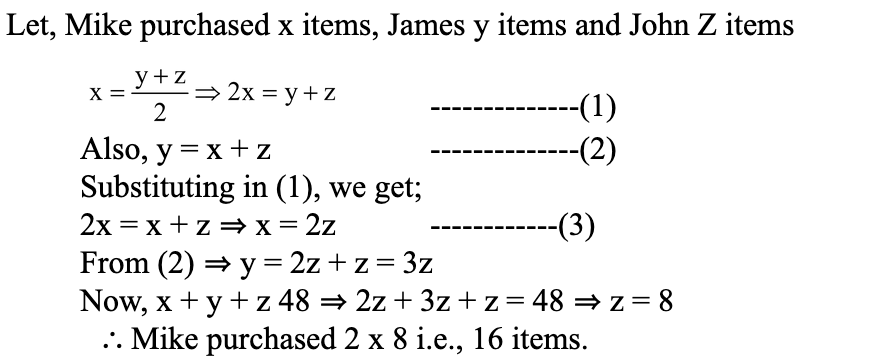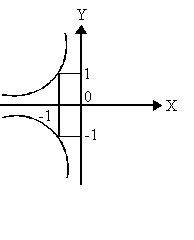XAT Quants Questions

## XAT Quants QuestionsJobs By Batch
Jobs By Location
Jobs By Degree
Jobs By Branch
IT Jobs
Internships
Govt. Jobs• ##### Original Questions of IT Companies| All Topics### Quiz Begins Here

Q #1
:

#### Quants Question

Directions: Read the following information to answer the question.

Three friends Mike, James and John went shopping. Mike purchased half as many items as were purchased by James and John together while James purchased as many items as were purchased by Mike and John together. If in all they purchased 48 items, how many items did Mike purchase?
+Q #2
:

#### Quants Question

Directions: Read the following information to answer the question.

In an examination, the maximum possible score is N while the pass mark is 45% of N. A candidate obtains 36 marks, but falls short of the pass mark by 68%. Which one of the following is then correct?
+

A got 36 marks but falls short of pass marks by 68%.

Maximum possible score is N.

Pass mark is 45% of N. 32% of 45% of N = 36 => N = 250

Q #3
:

#### Quants Question

Directions: Read the following information to answer the question.

A biologist catches a random sample of 60 fish from a lake, tags them and releases them. Six months later she catches a random sample of 70 fish and finds 3 are tagged. She assumes 25% of the fish in the lake on the earlier date have died or moved away and that 40% of the fish on the later date have arrived (or been born) since. What does she estimate as the number of fish in the lake on the earlier date?
+

Let no. of Fishes on earlier day = x
60 fishes are tagged, out of which 25% have died/moved
remaining fishes that are tagged = 45

No. of fishes on day of second observation = 5/4x
No. of fishes caught = 70
No. of Fishes tagged = 3 = 4.2857% (3/70)
Total No. fishes on Second day of Observation = 45/.042857 (45*70/3) = 1050

No of Fishes on earlier day = x = 4/5*1050 = 840

Q #4
:

#### Quants Question

Directions: Read the following information to answer the question.

Let p and q be the roots of the quadratic equation x2 � ( - 2) x - - 1 = 0. What is the minimum possible value of p2 + q2?
+

q = α–2 and pq = –α – 1

(p + q)2 = p2 + q2 + 2pq,

Thus (α–2)2 = p2 + q2 + 2(–α– 1)

p2 + q=  α2 – 4α + 4 + 2α + 2

p2 + q=  α2 – 2α + 6

p2 + q2 = α2 – 2α + 1 + 5

p2 + q2 = (α – 1)2 + 5

Thus, minimum value of p2 + q2 is 5.

Q #5
:

#### Quants Question

Directions: Read the following information to answer the question.

Identify the relation associated with the graph.+

 The graph lies in 2nd and 3rd consider x?y? = -1     ⇒ x = - 1/?y?. This is the only option which admits x negative.